Refer to our Texas Go Math Grade 2 Answer Key Pdf to score good marks in the exams. Test yourself by practicing the problems from Texas Go Math Grade 2 Lesson 6.3 Answer Key Break Apart Addends as Tens and Ones.

Explore

Write the number. Then write the number as tens plus ones.FOR THE TEACHER • Direct children’s attention to the orange box. Have children write 25 inside the large rectangle. Then ask children to write 25 as tens plus ones. Repeat the activity for 36 and 42.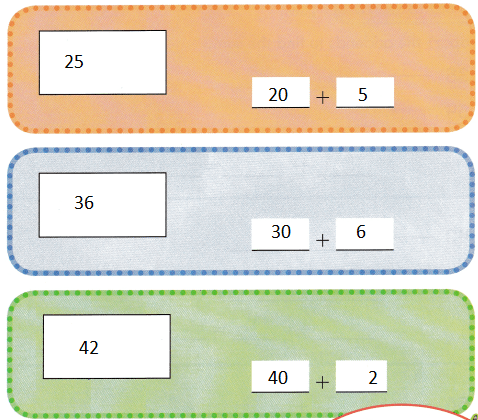Explanation:
25 is written as tens + ones
20 +5 = 25
30 + 6 = 36
40 + 2 = 42

Math Talk
Mathematical Processes

What is the value of the 6 in the number 63? Explain.
6 is in the tens place
so, the value of 6 is 60

Model and Draw

Break apart the addends into tens and ones. Add the tens. Add the ones. Find the sum.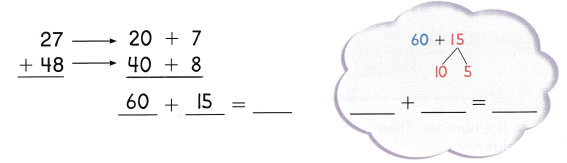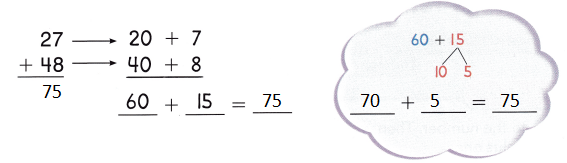Explanation:
75 is written as 70 + 5
7 tens and 5 ones

Share and Show

Break apart the addends to find the sum.

Question 1.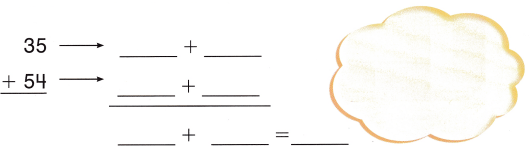Explanation:
tens and ones are added separately
80 + 9 = 89

Question 2.Explanation:
The tens and ones are added separately

Question 3.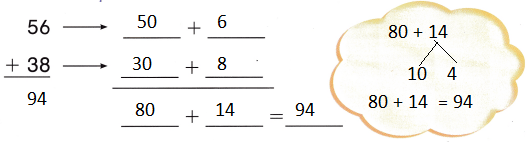Explanation:
The tens and ones are added separately
80 + 14 = 94
56 + 38 = 94

Problem Solving

Break apart the addends to find the sum.

Question 4.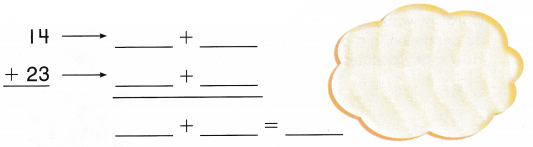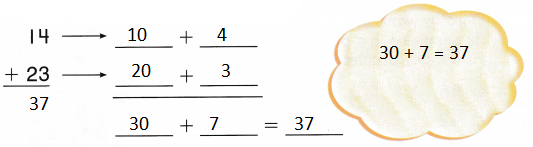Explanation:
The tens and ones are added separately
30 + 7 = 37
14 + 23 = 37

Question 5.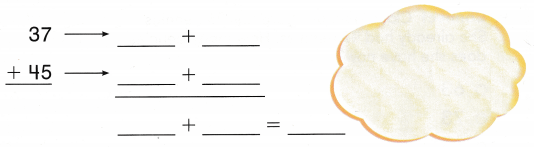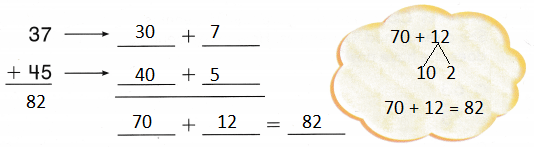Explanation:
The tens and ones are added separately
37 + 45 = 82
70 + 12 = 82

Solve. Write or draw to explain.

Question 6.
H.O.T. Multi-Step Julie read 18 pages of her book in the morning. She read the same number of pages in the afternoon. How many pages did she read?__________ pages
36 pages
Explanation:
Julie read 18 pages of her book in the morning.
She read the same number of pages in the afternoon.
18 + 18 =
20 + 16 =  36

Question 7.
H.O.T. Multi-Step Chris read 15 pages of his book. Tony read 4 more pages than Chris. How many pages did Chris and Tony read?___________ pages
34 pages
Explanation:
Chris read 15 pages of his book.
Tony read 4 more pages than Chris.
15 + 4 =
10 + 9 = 19
15 + 19 = 34
34  pages that Chris and Tony read

Question 8.
Dorothy wants to buy a stamp with 32 pennies and a postcard for 18 pennies. How many pennies would she need?(A) 46
(B) 42
(C) 50
Explanation:
Dorothy wants to buy a stamp with 32 pennies and a postcard for 18 pennies.
32 + 18 =
30 + 2  + 10 + 8 =
40 + 10 = 50
How many pennies would she need

Question 9.
Connect Claudia’s mom gave her 26 pennies. She already had 47 pennies. How many pennies does she have now?
(A) 63
(B) 73
(C) 68
Explanation:
Claudia’s mom gave her 26 pennies.
26 + 47 = 73
73 pennies that she have now

Question 10.
Analyze Mr. Green has 4-8 books on one shelf. He has 34- books on another shelf. How many books are on the two shelves?
(A) 84
(B) 70
(C) 82
Explanation:
Mr. Green has 4-8 books on one shelf.
He has 34- books on another shelf
48 + 34 = 82
82 books are on the two shelves

Question 11.
TEXAS Test Prep Tomas has 17 art pencils. He buys 26 more art pencils. How many art pencils does Tomas have now?(A) 43
(B) 33
(C) 41
Explanation:
Tomas has 17 art pencils.
He buys 26 more art pencils.
17 + 26 = 43
43 art pencils that Tomas have now

TAKE HOME ACTIVITY • Write 32 + 48 on a sheet of paper. Have your child break apart the numbers and find the sum.
32 + 48
30 + 2 + 40 + 8 =
70 + 10 = 80
32 + 48 = 80
Explanation:
The tens and ones are added seperately

Texas Go Math Grade 2 Lesson 6.3 Homework and Practice Answer Key

Break apart the addends to find the sum.

Question 1.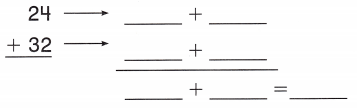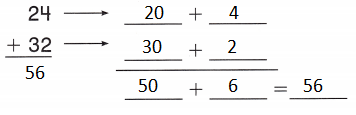Explanation:
The tens and ones are added separately
50 + 6 = 56
24 + 32 = 56

Question 2.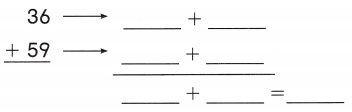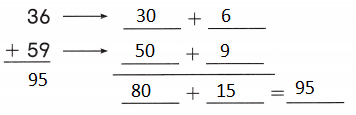Explanation:
The tens and ones are added separately
80 + 15 = 95
36 + 59 = 95

Question 3.Explanation:
The tens and ones are added separately
70 + 17 = 87
49 + 38 = 87

Problem Solving

Choose a way to solve. Write or draw to explain.

Question 4.
Multi-Step Emma found 12 acorns in the morning. She found 18 more acorns in the afternoon. Saul found 5 fewer acorns than Emma, How many acorns did Saul find?
___________ acorns
35 acorns
Emma found 12 acorns in the morning.
She found 18 more acorns in the afternoon.
Saul found 5 fewer acorns than Emma,
12 + 18 + 5 = 35
35 acorns that Saul find

Lesson Check

Question 5.
Ray has 19 marbles. He buys 35 more marbles. How many marbles does Ray have now?
(A) 54
(B) 44
(C) 55
Ray has 19 marbles.
19 + 35 =  54
54 marbles that Ray have now

Question 6.
(A) 70
(B) 69
(C) 79
Explanation:
Melinda found 42 beads in a bag today.
Then she finds 9 more beads.
42 + 28 + 9 = 79
79 beads that she have now

Question 7.
Julia counts two piles of pennies. There are 25 pennies in one pile and 69 pennies in the other. How many pennies does she have altogether?
(A) 84
(B) 94
(C) 95
Explanation:
Julia counts two piles of pennies.
There are 25 pennies in one pile and 69 pennies in the other.
25 + 69 = 94
94 pennies that she have altogether

Question 8.
Rick has 24 blue ribbons for swimming. He keeps them in an album. He also has 26 red ribbons for running. He keeps those ribbons in another album. How many ribbons are in the two albums?
(A) 42
(B) 40
(C) 50